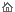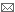FAQ'sHomeContactSearchPhotos of Me

How do I ... ?

[This is the answer to the question.]

Where can I find ... ?

[This is the answer to the question.]

Why doesn't ... ?

[This is the answer to the question.]

Who is ... ?

[This is the answer to the question.]

What is ... ?

[This is the answer to the question.]

When is ... ?

[This is the answer to the question.]